9 Linear Momentum and Collisions

# 9.3 Conservation of Linear Momentum

### Learning Objectives

By the end of this section, you will be able to:

• Explain the meaning of “conservation of momentum”
• Correctly identify if a system is, or is not, closed
• Define a system whose momentum is conserved
• Mathematically express conservation of momentum for a given system
• Calculate an unknown quantity using conservation of momentum

Recall Newton’s third law: When two objects of masses ${m}_{1}$ and ${m}_{2}$ interact (meaning that they apply forces on each other), the force that object 2 applies to object 1 is equal in magnitude and opposite in direction to the force that object 1 applies on object 2. Let:

• ${\mathbf{\overset{\to }{F}}}_{21}=$ the force on ${m}_{1}$ from ${m}_{2}$
• ${\mathbf{\overset{\to }{F}}}_{12}=$ the force on ${m}_{2}$ from ${m}_{1}$

Then, in symbols, Newton’s third law says

$\begin{array}{ccc}\hfill {\mathbf{\overset{\to }{F}}}_{21}& =\hfill & \text{−}{\mathbf{\overset{\to }{F}}}_{12}\hfill \\ \hfill {m}_{1}{\mathbf{\overset{\to }{a}}}_{1}& =\hfill & \text{−}{m}_{2}{\mathbf{\overset{\to }{a}}}_{2}.\hfill \end{array}$

(Recall that these two forces do not cancel because they are applied to different objects. ${F}_{21}$ causes ${m}_{1}$ to accelerate, and ${F}_{12}$ causes ${m}_{2}$ to accelerate.)

Although the magnitudes of the forces on the objects are the same, the accelerations are not, simply because the masses (in general) are different. Therefore, the changes in velocity of each object are different:

$\frac{d{\mathbf{\overset{\to }{v}}}_{1}}{dt}\ne \frac{d{\mathbf{\overset{\to }{v}}}_{2}}{dt}.$

However, the products of the mass and the change of velocity are equal (in magnitude):

${m}_{1}\frac{d{\mathbf{\overset{\to }{v}}}_{1}}{dt}=\text{−}{m}_{2}\frac{d{\mathbf{\overset{\to }{v}}}_{2}}{dt}.$

It’s a good idea, at this point, to make sure you’re clear on the physical meaning of the derivatives in Figure. Because of the interaction, each object ends up getting its velocity changed, by an amount dv. Furthermore, the interaction occurs over a time interval dt, which means that the change of velocities also occurs over dt. This time interval is the same for each object.

Let‘s assume, for the moment, that the masses of the objects do not change during the interaction. (We’ll relax this restriction later.) In that case, we can pull the masses inside the derivatives:

$\frac{d}{dt}({m}_{1}{\mathbf{\overset{\to }{v}}}_{1})=-\frac{d}{dt}({m}_{2}{\mathbf{\overset{\to }{v}}}_{2})$

and thus

$\frac{d{\mathbf{\overset{\to }{p}}}_{1}}{dt}=-\frac{d{\mathbf{\overset{\to }{p}}}_{2}}{dt}.$

This says that the rate at which momentum changes is the same for both objects. The masses are different, and the changes of velocity are different, but the rate of change of the product of m and $\mathbf{\overset{\to }{v}}$ are the same.

Physically, this means that during the interaction of the two objects (${m}_{1}\,\text{and}\,{m}_{2}$), both objects have their momentum changed; but those changes are identical in magnitude, though opposite in sign. For example, the momentum of object 1 might increase, which means that the momentum of object 2 decreases by exactly the same amount.

In light of this, let’s re-write Figure in a more suggestive form:

$\frac{d{\mathbf{\overset{\to }{p}}}_{1}}{dt}+\frac{d{\mathbf{\overset{\to }{p}}}_{2}}{dt}=0.$

This says that during the interaction, although object 1’s momentum changes, and object 2’s momentum also changes, these two changes cancel each other out, so that the total change of momentum of the two objects together is zero.

Since the total combined momentum of the two objects together never changes, then we could write

$\frac{d}{dt}({\mathbf{\overset{\to }{p}}}_{1}+{\mathbf{\overset{\to }{p}}}_{2})=0$

from which it follows that

${\mathbf{\overset{\to }{p}}}_{1}+{\mathbf{\overset{\to }{p}}}_{2}=\text{constant}.$

As shown in Figure, the total momentum of the system before and after the collision remains the same.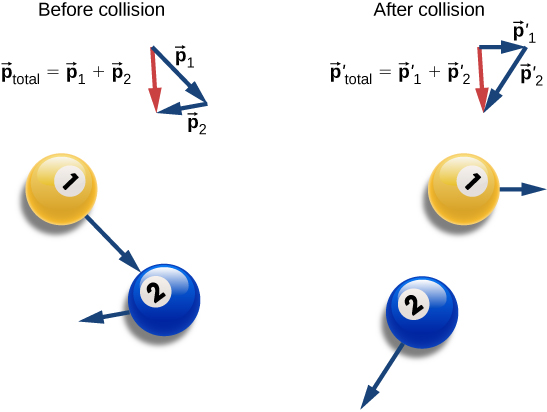Figure 9.14 Before the collision, the two billiard balls travel with momenta ${\mathbf{\overset{\to }{p}}}_{1}$ and ${\mathbf{\overset{\to }{p}}}_{3}$. The total momentum of the system is the sum of these, as shown by the red vector labeled ${\mathbf{\overset{\to }{p}}}_{\mathrm{total}}$ on the left. After the collision, the two billiard balls travel with different momenta ${{\mathbf{\overset{\to }{p}}}^{\prime }}_{1}$ and ${{\mathbf{\overset{\to }{p}}}^{\prime }}_{3}$. The total momentum, however, has not changed, as shown by the red vector arrow ${{\mathbf{\overset{\to }{p}}}^{\prime }}_{\mathrm{total}}$ on the right.

Generalizing this result to N objects, we obtain

$\begin{array}{ccc}\hfill {\mathbf{\overset{\to }{p}}}_{1}+{\mathbf{\overset{\to }{p}}}_{2}+{\mathbf{\overset{\to }{p}}}_{3}+\cdots +{\mathbf{\overset{\to }{p}}}_{N}& =\hfill & \text{constant}\hfill \\ \hfill \sum _{j=1}^{N}{\mathbf{\overset{\to }{p}}}_{j}& =\hfill & \text{constant.}\hfill \end{array}$

Figure is the definition of the total (or net) momentum of a system of N interacting objects, along with the statement that the total momentum of a system of objects is constant in time—or better, is conserved.

### Conservation Laws

If the value of a physical quantity is constant in time, we say that the quantity is conserved.

### Requirements for Momentum Conservation

There is a complication, however. A system must meet two requirements for its momentum to be conserved:

1. The mass of the system must remain constant during the interaction.As the objects interact (apply forces on each other), they may transfer mass from one to another; but any mass one object gains is balanced by the loss of that mass from another. The total mass of the system of objects, therefore, remains unchanged as time passes:
${[\frac{dm}{dt}]}_{\text{system}}=0.$
2. The net external force on the system must be zero.As the objects collide, or explode, and move around, they exert forces on each other. However, all of these forces are internal to the system, and thus each of these internal forces is balanced by another internal force that is equal in magnitude and opposite in sign. As a result, the change in momentum caused by each internal force is cancelled by another momentum change that is equal in magnitude and opposite in direction. Therefore, internal forces cannot change the total momentum of a system because the changes sum to zero. However, if there is some external force that acts on all of the objects (gravity, for example, or friction), then this force changes the momentum of the system as a whole; that is to say, the momentum of the system is changed by the external force. Thus, for the momentum of the system to be conserved, we must have
${\mathbf{\overset{\to }{F}}}_{ext}=\mathbf{\overset{\to }{0}}.$

A system of objects that meets these two requirements is said to be a closed system (also called an isolated system). Thus, the more compact way to express this is shown below.

#### Law of Conservation of Momentum

The total momentum of a closed system is conserved:

$\sum _{j=1}^{N}{\mathbf{\overset{\to }{p}}}_{j}=\text{constant.}$

This statement is called the Law of Conservation of Momentum. Along with the conservation of energy, it is one of the foundations upon which all of physics stands. All our experimental evidence supports this statement: from the motions of galactic clusters to the quarks that make up the proton and the neutron, and at every scale in between. In a closed system, the total momentum never changes.

Note that there absolutely can be external forces acting on the system; but for the system’s momentum to remain constant, these external forces have to cancel, so that the net external force is zero. Billiard balls on a table all have a weight force acting on them, but the weights are balanced (canceled) by the normal forces, so there is no net force.

### The Meaning of ‘System’

A system (mechanical) is the collection of objects in whose motion (kinematics and dynamics) you are interested. If you are analyzing the bounce of a ball on the ground, you are probably only interested in the motion of the ball, and not of Earth; thus, the ball is your system. If you are analyzing a car crash, the two cars together compose your system (Figure).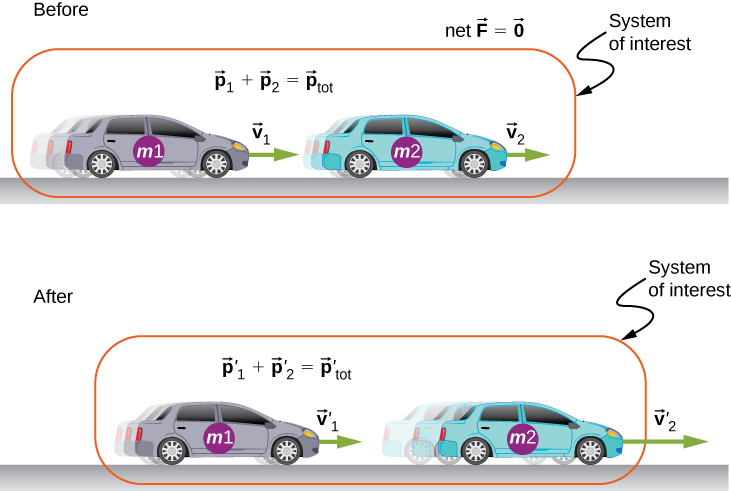Figure 9.15 The two cars together form the system that is to be analyzed. It is important to remember that the contents (the mass) of the system do not change before, during, or after the objects in the system interact.

#### Problem-Solving Strategy: Conservation of Momentum

Using conservation of momentum requires four basic steps. The first step is crucial:

1. Identify a closed system (total mass is constant, no net external force acts on the system).
2. Write down an expression representing the total momentum of the system before the “event” (explosion or collision).
3. Write down an expression representing the total momentum of the system after the “event.”
4. Set these two expressions equal to each other, and solve this equation for the desired quantity.

### Example

#### Colliding Carts

Two carts in a physics lab roll on a level track, with negligible friction. These carts have small magnets at their ends, so that when they collide, they stick together (Figure). The first cart has a mass of 675 grams and is rolling at 0.75 m/s to the right; the second has a mass of 500 grams and is rolling at 1.33 m/s, also to the right. After the collision, what is the velocity of the two joined carts?

#### Strategy

We have a collision. We’re given masses and initial velocities; we’re asked for the final velocity. This all suggests using conservation of momentum as a method of solution. However, we can only use it if we have a closed system. So we need to be sure that the system we choose has no net external force on it, and that its mass is not changed by the collision.

Defining the system to be the two carts meets the requirements for a closed system: The combined mass of the two carts certainly doesn’t change, and while the carts definitely exert forces on each other, those forces are internal to the system, so they do not change the momentum of the system as a whole. In the vertical direction, the weights of the carts are canceled by the normal forces on the carts from the track.

#### Solution

Conservation of momentum is

${\mathbf{\overset{\to }{p}}}_{f}={\mathbf{\overset{\to }{p}}}_{i}.$

Define the direction of their initial velocity vectors to be the +x-direction. The initial momentum is then

${\mathbf{\overset{\to }{p}}}_{i}={m}_{1}{v}_{1}\mathbf{\hat{i}}+{m}_{2}{v}_{2}\mathbf{\hat{i}}.$

The final momentum of the now-linked carts is

${\mathbf{\overset{\to }{p}}}_{f}=({m}_{1}+{m}_{2}){\mathbf{\overset{\to }{v}}}_{f}.$

Equating:

$\begin{array}{ccc}\hfill ({m}_{1}+{m}_{2}){\mathbf{\overset{\to }{v}}}_{f}& =\hfill & {m}_{1}{v}_{1}\mathbf{\hat{i}}+{m}_{2}{v}_{2}\mathbf{\hat{i}}\hfill \\ \hfill {\mathbf{\overset{\to }{v}}}_{f}& =\hfill & (\frac{{m}_{1}{v}_{1}+{m}_{2}{v}_{2}}{{m}_{1}+{m}_{2}})\mathbf{\hat{i}}.\hfill \end{array}$

Substituting the given numbers:

$\begin{array}{cc}\hfill {\mathbf{\overset{\to }{v}}}_{f}& =[\frac{(0.675\,\text{kg})(0.75\,\text{m/s})+(0.5\,\text{kg})(1.33\,\text{m/s})}{1.175\,\text{kg}}]\mathbf{\hat{i}}\hfill \\ & =(0.997\,\text{m/s})\mathbf{\hat{i}}.\hfill \end{array}$

#### Significance

The principles that apply here to two laboratory carts apply identically to all objects of whatever type or size. Even for photons, the concepts of momentum and conservation of momentum are still crucially important even at that scale. (Since they are massless, the momentum of a photon is defined very differently from the momentum of ordinary objects. You will learn about this when you study quantum physics.)

Suppose the second, smaller cart had been initially moving to the left. What would the sign of the final velocity have been in this case?

Show Solution

If the smaller cart were rolling at 1.33 m/s to the left, then conservation of momentum gives

$\begin{array}{ccc}\hfill ({m}_{1}+{m}_{2}){\mathbf{\overset{\to }{v}}}_{f}& =\hfill & {m}_{1}{v}_{1}\mathbf{\hat{i}}-{m}_{2}{v}_{2}\mathbf{\hat{i}}\hfill \\ \hfill {\mathbf{\overset{\to }{v}}}_{f}& =\hfill & (\frac{{m}_{1}{v}_{1}-{m}_{2}{v}_{2}}{{m}_{1}+{m}_{2}})\mathbf{\hat{i}}\hfill \\ & =\hfill & [\frac{(0.675\,\text{kg})(0.75\,\text{m/s})-(0.500\,\text{kg})(1.33\,\text{m/s})}{1.175\,\text{kg}}]\mathbf{\hat{i}}\hfill \\ & =\hfill & \text{−}(0.135\,\text{m/s})\mathbf{\hat{i}}\hfill \end{array}$

Thus, the final velocity is 0.135 m/s to the left.

### Example

#### A Bouncing Superball

A superball of mass 0.25 kg is dropped from rest from a height of $h=1.50\,\text{m}$ above the floor. It bounces with no loss of energy and returns to its initial height (Figure).

1. What is the superball’s change of momentum during its bounce on the floor?
2. What was Earth’s change of momentum due to the ball colliding with the floor?
3. What was Earth’s change of velocity as a result of this collision?

(This example shows that you have to be careful about defining your system.)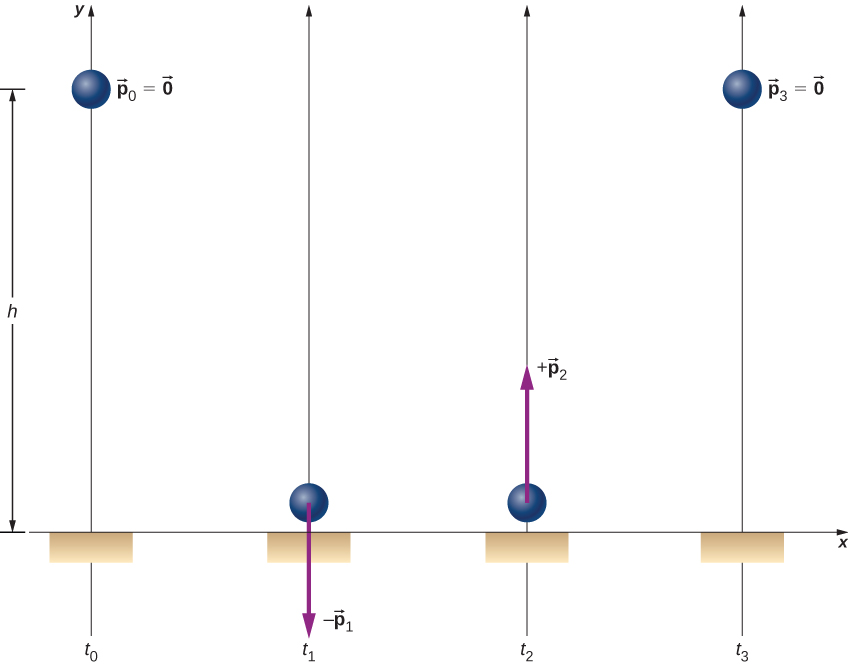Figure 9.17 A superball is dropped to the floor (${t}_{0}$), hits the floor (${t}_{1}$), bounces (${t}_{2}$), and returns to its initial height (${t}_{3}$).

#### Strategy

Since we are asked only about the ball’s change of momentum, we define our system to be the ball. But this is clearly not a closed system; gravity applies a downward force on the ball while it is falling, and the normal force from the floor applies a force during the bounce. Thus, we cannot use conservation of momentum as a strategy. Instead, we simply determine the ball’s momentum just before it collides with the floor and just after, and calculate the difference. We have the ball’s mass, so we need its velocities.

#### Solution

1. Since this is a one-dimensional problem, we use the scalar form of the equations. Let:
• ${p}_{0}=$ the magnitude of the ball’s momentum at time ${t}_{0}$, the moment it was released; since it was dropped from rest, this is zero.
• ${p}_{1}=$ the magnitude of the ball’s momentum at time ${t}_{1}$, the instant just before it hits the floor.
• ${p}_{2}=$ the magnitude of the ball’s momentum at time ${t}_{2}$, just after it loses contact with the floor after the bounce.

The ball’s change of momentum is

$\begin{array}{cc}\hfill \Delta \mathbf{\overset{\to }{p}}& ={\mathbf{\overset{\to }{p}}}_{2}-{\mathbf{\overset{\to }{p}}}_{1}\hfill \\ & ={p}_{2}\mathbf{\hat{j}}-(\text{−}{p}_{1}\mathbf{\hat{j}})\hfill \\ & =({p}_{2}+{p}_{1})\mathbf{\hat{j}}.\hfill \end{array}$

Its velocity just before it hits the floor can be determined from either conservation of energy or kinematics. We use kinematics here; you should re-solve it using conservation of energy and confirm you get the same result.

We want the velocity just before it hits the ground (at time ${t}_{1}$). We know its initial velocity ${v}_{0}=0$ (at time ${t}_{0}$), the height it falls, and its acceleration; we don’t know the fall time. We could calculate that, but instead we use

${\mathbf{\overset{\to }{v}}}_{1}=\text{−}\mathbf{\hat{j}}\sqrt{2gy}=-5.4\,\text{m/s}\mathbf{\hat{j}}.$

Thus the ball has a momentum of

$\begin{array}{cc}\hfill {\mathbf{\overset{\to }{p}}}_{1}& =\text{−}(0.25\,\text{kg})(-5.4\,\text{m/s}\mathbf{\hat{j}})\hfill \\ & =\text{−}(1.4\,\text{kg}\cdot \text{m/s})\mathbf{\hat{j}}.\hfill \end{array}$

We don’t have an easy way to calculate the momentum after the bounce. Instead, we reason from the symmetry of the situation.

Before the bounce, the ball starts with zero velocity and falls 1.50 m under the influence of gravity, achieving some amount of momentum just before it hits the ground. On the return trip (after the bounce), it starts with some amount of momentum, rises the same 1.50 m it fell, and ends with zero velocity. Thus, the motion after the bounce was the mirror image of the motion before the bounce. From this symmetry, it must be true that the ball’s momentum after the bounce must be equal and opposite to its momentum before the bounce. (This is a subtle but crucial argument; make sure you understand it before you go on.)

Therefore,

${\mathbf{\overset{\to }{p}}}_{2}=\text{−}{\mathbf{\overset{\to }{p}}}_{1}=+(1.4\,\text{kg}\cdot \text{m/s})\mathbf{\hat{j}}.$

Thus, the ball’s change of velocity during the bounce is

$\begin{array}{cc}\hfill \Delta \mathbf{\overset{\to }{p}}& ={\mathbf{\overset{\to }{p}}}_{2}-{\mathbf{\overset{\to }{p}}}_{1}\hfill \\ & =(1.4\,\text{kg}\cdot \text{m/s})\mathbf{\hat{j}}-(-1.4\,\text{kg}\cdot \text{m/s})\mathbf{\hat{j}}\hfill \\ & =+(2.8\,\text{kg}\cdot \text{m/s})\mathbf{\hat{j}}.\hfill \end{array}$
2. What was Earth’s change of momentum due to the ball colliding with the floor?Your instinctive response may well have been either “zero; the Earth is just too massive for that tiny ball to have affected it” or possibly, “more than zero, but utterly negligible.” But no—if we re-define our system to be the Superball + Earth, then this system is closed (neglecting the gravitational pulls of the Sun, the Moon, and the other planets in the solar system), and therefore the total change of momentum of this new system must be zero. Therefore, Earth’s change of momentum is exactly the same magnitude:
$\Delta {\mathbf{\overset{\to }{p}}}_{\text{Earth}}=-2.8\,\text{kg}\cdot \text{m/s}\mathbf{\hat{j}}.$
3. What was Earth’s change of velocity as a result of this collision?This is where your instinctive feeling is probably correct:
$\begin{array}{cc}\hfill \Delta {\mathbf{\overset{\to }{v}}}_{\text{Earth}}& =\frac{\Delta {\mathbf{\overset{\to }{p}}}_{\text{Earth}}}{{M}_{\text{Earth}}}\hfill \\ & =-\frac{2.8\,\text{kg}\cdot \text{m/s}}{5.97\times {10}^{24}\,\text{kg}}\mathbf{\hat{j}}\hfill \\ & =\text{−}(4.7\times {10}^{-25}\,\text{m/s})\mathbf{\hat{j}}.\hfill \end{array}$

This change of Earth’s velocity is utterly negligible.

#### Significance

It is important to realize that the answer to part (c) is not a velocity; it is a change of velocity, which is a very different thing. Nevertheless, to give you a feel for just how small that change of velocity is, suppose you were moving with a velocity of $4.7\times {10}^{-25}\,\text{m/s}$. At this speed, it would take you about 7 million years to travel a distance equal to the diameter of a hydrogen atom.

Would the ball’s change of momentum have been larger, smaller, or the same, if it had collided with the floor and stopped (without bouncing)?

Would the ball’s change of momentum have been larger, smaller, or the same, if it had collided with the floor and stopped (without bouncing)?

Show Solution

If the ball does not bounce, its final momentum ${\mathbf{\overset{\to }{p}}}_{2}$ is zero, so $\begin{array}{cc}\hfill \Delta \mathbf{\overset{\to }{p}}& ={\mathbf{\overset{\to }{p}}}_{2}-{\mathbf{\overset{\to }{p}}}_{1}\hfill \\ & =(0)\mathbf{\hat{j}}-(-1.4\,\text{kg}\cdot \text{m/s})\mathbf{\hat{j}}\hfill \\ & =+(1.4\,\text{kg}\cdot \text{m/s})\mathbf{\hat{j}}\hfill \end{array}$

### Example

#### Ice Hockey 1

Two hockey pucks of identical mass are on a flat, horizontal ice hockey rink. The red puck is motionless; the blue puck is moving at 2.5 m/s to the left (Figure). It collides with the motionless red puck. The pucks have a mass of 15 g. After the collision, the red puck is moving at 2.5 m/s, to the left. What is the final velocity of the blue puck?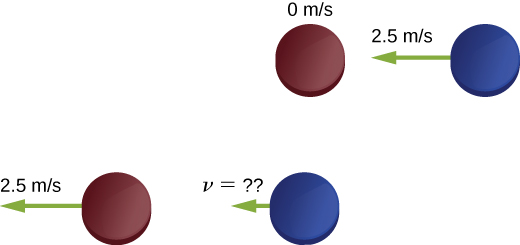Figure 9.18 Two identical hockey pucks colliding. The top diagram shows the pucks the instant before the collision, and the bottom diagram show the pucks the instant after the collision. The net external force is zero.

#### Strategy

We’re told that we have two colliding objects, we’re told the masses and initial velocities, and one final velocity; we’re asked for both final velocities. Conservation of momentum seems like a good strategy. Define the system to be the two pucks; there’s no friction, so we have a closed system.

Before you look at the solution, what do you think the answer will be?

The blue puck final velocity will be:

• zero
• 2.5 m/s to the left
• 2.5 m/s to the right
• 1.25 m/s to the left
• 1.25 m/s to the right
• something else

#### Solution

Define the +x-direction to point to the right. Conservation of momentum then reads

$\begin{array}{ccc}\hfill {\mathbf{\overset{\to }{p}}}_{f}& =\hfill & {\mathbf{\overset{\to }{p}}}_{i}\hfill \\ \hfill m{v}_{{\text{r}}_{\text{f}}}\mathbf{\hat{i}}+m{v}_{{\text{b}}_{\text{f}}}\mathbf{\hat{i}}& =\hfill & m{v}_{{\text{r}}_{\text{i}}}\mathbf{\hat{i}}-m{v}_{{\text{b}}_{\text{i}}}\mathbf{\hat{i}}.\hfill \end{array}$

Before the collision, the momentum of the system is entirely and only in the blue puck. Thus,

$\begin{array}{ccc}\hfill m{v}_{{\text{r}}_{\text{f}}}\mathbf{\hat{i}}+m{v}_{{\text{b}}_{\text{f}}}\mathbf{\hat{i}}& =\hfill & \text{−}m{v}_{{\text{b}}_{\text{i}}}\mathbf{\hat{i}}\hfill \\ \hfill {v}_{{\text{r}}_{\text{f}}}\mathbf{\hat{i}}+{v}_{{\text{b}}_{\text{f}}}\mathbf{\hat{i}}& =\hfill & \text{−}{v}_{{\text{b}}_{\text{i}}}\mathbf{\hat{i}}.\hfill \end{array}$

(Remember that the masses of the pucks are equal.) Substituting numbers:

$\begin{array}{ccc}\hfill -(2.5\,\text{m/s})\mathbf{\hat{i}}+{\mathbf{\overset{\to }{v}}}_{{\text{b}}_{\text{f}}}& =\hfill & \text{−}(2.5\,\text{m/s})\mathbf{\hat{i}}\hfill \\ \hfill {\mathbf{\overset{\to }{v}}}_{{\text{b}}_{\text{f}}}& =\hfill & 0.\hfill \end{array}$

#### Significance

Evidently, the two pucks simply exchanged momentum. The blue puck transferred all of its momentum to the red puck. In fact, this is what happens in similar collision where ${m}_{1}={m}_{2}.$

Even if there were some friction on the ice, it is still possible to use conservation of momentum to solve this problem, but you would need to impose an additional condition on the problem. What is that additional condition?

Show Solution

Consider the impulse momentum theory, which is $\mathbf{\overset{\to }{J}}=\Delta \mathbf{\overset{\to }{p}}$. If $\mathbf{\overset{\to }{J}}=0$, we have the situation described in the example. If a force acts on the system, then $\mathbf{\overset{\to }{J}}={\mathbf{\overset{\to }{F}}}_{\text{ave}}\Delta t$. Thus, instead of ${\mathbf{\overset{\to }{p}}}_{f}={\mathbf{\overset{\to }{p}}}_{i}$, we have

${\mathbf{\overset{\to }{F}}}_{\text{ave}}\Delta t=\Delta \mathbf{\overset{\to }{p}}={\mathbf{\overset{\to }{p}}}_{\text{f}}-{\mathbf{\overset{\to }{p}}}_{\text{i}}$

where ${\mathbf{\overset{\to }{F}}}_{\text{ave}}$ is the force due to friction.

### Example

#### Landing of Philae

On November 12, 2014, the European Space Agency successfully landed a probe named Philae on Comet 67P/Churyumov/Gerasimenko (Figure). During the landing, however, the probe actually landed three times, because it bounced twice. Let’s calculate how much the comet’s speed changed as a result of the first bounce.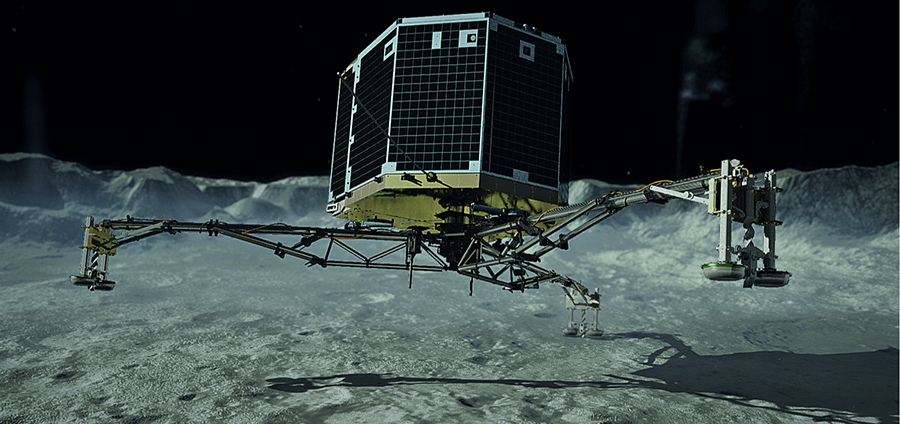Figure 9.19 An artist’s rendering of Philae landing on a comet. (credit: modification of work by “DLR German Aerospace Center”/Flickr)

Let’s define upward to be the +y-direction, perpendicular to the surface of the comet, and $y=0$ to be at the surface of the comet. Here’s what we know:

• The mass of Comet 67P: ${M}_{c}=1.0\times {10}^{13}\,\text{kg}$
• The acceleration due to the comet’s gravity: $\mathbf{\overset{\to }{a}}=\text{−}(5.0\times {10}^{-3}\,{\text{m/s}}^{2})\mathbf{\hat{j}}$
• Philae’s mass: ${M}_{p}=96\,\text{kg}$
• Initial touchdown speed: ${\mathbf{\overset{\to }{v}}}_{1}=\text{−}(1.0\,\text{m/s})\mathbf{\hat{j}}$
• Initial upward speed due to first bounce: ${\mathbf{\overset{\to }{v}}}_{2}=(0.38\,\text{m/s})\mathbf{\hat{j}}$
• Landing impact time: $\Delta t=1.3\,\text{s}$

#### Strategy

We’re asked for how much the comet’s speed changed, but we don’t know much about the comet, beyond its mass and the acceleration its gravity causes. However, we are told that the Philae lander collides with (lands on) the comet, and bounces off of it. A collision suggests momentum as a strategy for solving this problem.

If we define a system that consists of both Philae and Comet 67/P, then there is no net external force on this system, and thus the momentum of this system is conserved. (We’ll neglect the gravitational force of the sun.) Thus, if we calculate the change of momentum of the lander, we automatically have the change of momentum of the comet. Also, the comet’s change of velocity is directly related to its change of momentum as a result of the lander “colliding” with it.

#### Solution

Let ${\mathbf{\overset{\to }{p}}}_{1}$ be Philae’s momentum at the moment just before touchdown, and ${\mathbf{\overset{\to }{p}}}_{2}$ be its momentum just after the first bounce. Then its momentum just before landing was

${\mathbf{\overset{\to }{p}}}_{1}={M}_{p}{\mathbf{\overset{\to }{v}}}_{1}=(96\,\text{kg})(\text{−}1.0\,\text{m/s}\mathbf{\hat{j}})=\text{−}(96\,\text{kg}\cdot \text{m/s})\mathbf{\hat{j}}$

and just after was

${\mathbf{\overset{\to }{p}}}_{2}={M}_{p}{\mathbf{\overset{\to }{v}}}_{2}=(96\,\text{kg})(+0.38\,\text{m/s}\mathbf{\hat{j}})=(36.5\,\text{kg}\cdot \text{m/s})\mathbf{\hat{j}}.$

Therefore, the lander’s change of momentum during the first bounce is

$\begin{array}{c}\Delta \mathbf{\overset{\to }{p}}={\mathbf{\overset{\to }{p}}}_{2}-{\mathbf{\overset{\to }{p}}}_{1}\hfill \\ & =(36.5\,\text{kg}\cdot \text{m/s})\mathbf{\hat{j}}-(-96.0\,\text{kg}\cdot \text{m/s}\mathbf{\hat{j}})=(133\,\text{kg}\cdot \text{m/s})\mathbf{\hat{j}}\hfill \end{array}$

Notice how important it is to include the negative sign of the initial momentum.

Now for the comet. Since momentum of the system must be conserved, the comet’s momentum changed by exactly the negative of this:

$\Delta {\mathbf{\overset{\to }{p}}}_{c}=\text{−}\Delta \mathbf{\overset{\to }{p}}=\text{−}(133\,\text{kg}\cdot \text{m/s})\mathbf{\hat{j}}.$

Therefore, its change of velocity is

$\Delta {\mathbf{\overset{\to }{v}}}_{c}=\frac{\Delta {\mathbf{\overset{\to }{p}}}_{c}}{{M}_{c}}=\frac{\text{−}(133\,\text{kg}\cdot \text{m/s})\mathbf{\hat{j}}}{1.0\times {10}^{13}\,\text{kg}}=\text{−}(1.33\times {10}^{-11}\,\text{m/s})\mathbf{\hat{j}}.$

#### Significance

This is a very small change in velocity, about a thousandth of a billionth of a meter per second. Crucially, however, it is not zero.

The changes of momentum for Philae and for Comet 67/P were equal (in magnitude). Were the impulses experienced by Philae and the comet equal? How about the forces? How about the changes of kinetic energies?

Show Solution

The impulse is the change in momentum multiplied by the time required for the change to occur. By conservation of momentum, the changes in momentum of the probe and the comment are of the same magnitude, but in opposite directions, and the interaction time for each is also the same. Therefore, the impulse each receives is of the same magnitude, but in opposite directions. Because they act in opposite directions, the impulses are not the same. As for the impulse, the force on each body acts in opposite directions, so the forces on each are not equal. However, the change in kinetic energy differs for each, because the collision is not elastic.

### Summary

• The law of conservation of momentum says that the momentum of a closed system is constant in time (conserved).
• A closed (or isolated) system is defined to be one for which the mass remains constant, and the net external force is zero.
• The total momentum of a system is conserved only when the system is closed.

### Conceptual Questions

Under what circumstances is momentum conserved?

Show Solution

Momentum is conserved when the mass of the system of interest remains constant during the interaction in question and when no net external force acts on the system during the interaction.

Can momentum be conserved for a system if there are external forces acting on the system? If so, under what conditions? If not, why not?

Explain in terms of momentum and Newton’s laws how a car’s air resistance is due in part to the fact that it pushes air in its direction of motion.

Show Solution

To accelerate air molecules in the direction of motion of the car, the car must exert a force on these molecules by Newton’s second law $\mathbf{\overset{\to }{F}}=d\mathbf{\overset{\to }{p}}\text{/}dt$. By Newton’s third law, the air molecules exert a force of equal magnitude but in the opposite direction on the car. This force acts in the direction opposite the motion of the car and constitutes the force due to air resistance.

Can objects in a system have momentum while the momentum of the system is zero? Explain your answer.

A sprinter accelerates out of the starting blocks. Can you consider him as a closed system? Explain.

Show Solution

No, he is not a closed system because a net nonzero external force acts on him in the form of the starting blocks pushing on his feet.

A rocket in deep space (zero gravity) accelerates by firing hot gas out of its thrusters. Does the rocket constitute a closed system? Explain.

### Problems

Train cars are coupled together by being bumped into one another. Suppose two loaded train cars are moving toward one another, the first having a mass of $1.50\times {10}^{5}\,\text{kg}$ and a velocity of $(0.30\,\text{m/s})\mathbf{\hat{i}}$, and the second having a mass of $1.10\times {10}^{5}\,\text{kg}$ and a velocity of $\text{−}(0.12\,\text{m/s})\mathbf{\hat{i}}$. What is their final velocity?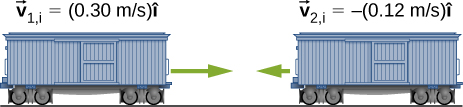$(0.122\,\text{m/s})\mathbf{\hat{i}}$

Two identical pucks collide elastically on an air hockey table. Puck 1 was originally at rest; puck 2 has an incoming speed of 6.00 m/s and scatters at an angle of $30^\circ$ with respect to its incoming direction. What is the velocity (magnitude and direction) of puck 1 after the collision?The figure below shows a bullet of mass 200 g traveling horizontally towards the east with speed 400 m/s, which strikes a block of mass 1.5 kg that is initially at rest on a frictionless table.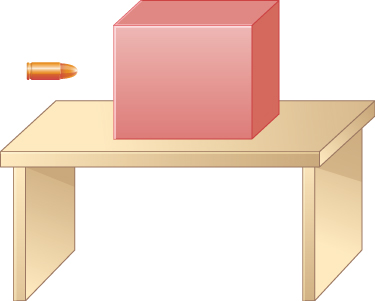After striking the block, the bullet is embedded in the block and the block and the bullet move together as one unit.

1. What is the magnitude and direction of the velocity of the block/bullet combination immediately after the impact?
2. What is the magnitude and direction of the impulse by the block on the bullet?
3. What is the magnitude and direction of the impulse from the bullet on the block?
4. If it took 3 ms for the bullet to change the speed from 400 m/s to the final speed after impact, what is the average force between the block and the bullet during this time?

a. 47 m/s in the bullet to block direction; b.$70.6\,\text{N}\cdot \text{s}$, toward the bullet; c. $70.6\,\text{N}\cdot \text{s}$, toward the block; d. magnitude is $2.35\times {10}^{4}\,\text{N}$

A 20-kg child is coasting at 3.3 m/s over flat ground in a 4.0-kg wagon. The child drops a 1.0-kg ball out the back of the wagon. What is the final speed of the child and wagon?

A 5000-kg paving truck (mass excluding the gravel) coasts over a road at 2.5 m/s and quickly dumps 1000 kg of gravel on the road backward at 0.5 m/s. What is the speed of the truck after dumping the gravel?

Show Solution

3.1 m/s

Explain why a cannon recoils when it fires a shell.

Two figure skaters are coasting in the same direction, with the leading skater moving at 5.5 m/s and the trailing skating moving at 6.2 m/s. When the trailing skater catches up with the leading skater, he picks her up without applying any horizontal forces on his skates. If the trailing skater is 50% heavier than the 50-kg leading skater, what is their speed after he picks her up?

Show Solution

5.9 m/s

A 2000-kg railway freight car coasts at 4.4 m/s underneath a grain terminal, which dumps grain directly down into the freight car. If the speed of the loaded freight car must not go below 3.0 m/s, what is the maximum mass of grain that it can accept?

### Glossary

closed system
system for which the mass is constant and the net external force on the system is zero
Law of Conservation of Momentum
total momentum of a closed system cannot change
system
object or collection of objects whose motion is currently under investigation; however, your system is defined at the start of the problem, you must keep that definition for the entire problem